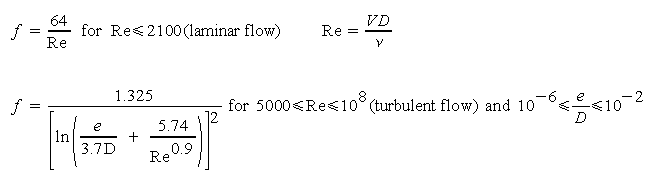Moody Friction Factor Calculator

Calculation uses an equation that simulates the Moody Diagram.  Turbulent or laminar flow.

 Select Calculation: Circular Duct: Enter D and Q Kinematic viscosity, ν (L2/T): Circular Duct: Enter D and V Surface Roughness, e (L): Circular Duct: Enter D and Re Duct Diameter, D (L): Non-circular Duct: Enter A, P, and Q Duct Area, A (L2): Non-circular Duct: Enter A, P, and V Duct Perimeter, P (L): Non-circular Duct: Enter A, P, and Re Velocity, V (L/T): © 2014 LMNO Engineering, Discharge, Q (L3/T): Research, and Software, Ltd. Reynolds Number: http://www.LMNOeng.com e/D: Moody Friction Factor, f:D = Diameter of a circular duct. If duct is non-circular, then D is computed as the hydraulic diameter of a rectangular duct, where D = 4A / P  per our non-circular duct page.
Re = Reynolds Number. The symbol Re is not the product (R)(e).

The equations used in this program represent the Moody diagram which is the old-fashioned way of finding f. You may enter numbers in any units, so long as you are consistent. (L) means that the variable has units of length (e.g. meters). (L3/T) means that the variable has units of cubic length per time (e.g. m3/s). The Moody friction factor (f) is used in the Darcy-Weisbach major loss equation. Note that for laminar flow, f is independent of e. However, you must still enter an e for the program to run even though e is not used to compute f. Equations can be found in Discussion and References for Closed Conduit Flow.

A more complicated equation which represents a slightly larger range of Reynolds numbers and e/D's is used in Design of Circular Liquid or Gas Pipes.

© 1999-2014 LMNO Engineering, Research, and Software, Ltd.  All rights reserved.

LMNO Engineering, Research, and Software, Ltd.
7860 Angel Ridge Rd.   Athens, Ohio 45701  USA   Phone: (740) 592-1890
LMNO@LMNOeng.com    http://www.LMNOeng.com

To:
LMNO Engineering home page (more calculations)

Related calculations:

Unit Conversions

Design of Circular Liquid or Gas Pipes Calculator